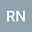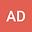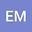Identification of collective particle motion in a rotating drum using a graph community detection algorithm
•••• +1Edgaras Misiulis
Lithuanian Energy Institute
Author ProfileGediminas Skarbalius
Lithuanian Energy Institute
Author Profile## Abstract

We present the method for detection of particle groups involved in collective motion based on network analysis. Knowing the positions and velocities of individual particles, a “velocity similarity graph’‘ is built, where the graph vertices represent the particles. The vertex pairs are connected by the edge if the distance between the respective particles is small enough. The edge weight is calculated to be inversely proportional to the difference in the respective particle velocities, i.e., the vertex pairs representing nearby particles having similar velocities are connected by edges of larger weight. If a group of particles moves in a coordinated matter, the particles in this group will have similar velocities, therefore, the corresponding vertices in the graph will be connected by edges of larger weight in the representing graph. Having produced the velocity similarity graph, identification of particle groups becomes equivalent to the problem of “community detection” in graph analysis. The algorithms and techniques developed for community detection in graphs can be thereby applied for identification of particle groups involved in coordinated motion in granular matter. We illustrate this approach by an example of granular media filled in a rotating cylinder.
31 Dec 2020Submitted to Mathematical Methods in the Applied Sciences
07 Jan 2021Submission Checks Completed
07 Jan 2021Assigned to Editor
10 Jan 2021Reviewer(s) Assigned
15 Mar 2021Review(s) Completed, Editorial Evaluation Pending
11 Apr 2021Editorial Decision: Revise Major